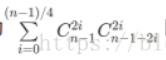# Wannafly 挑战赛 18-序列

64bit IO Format: %lld

#### 示例 1

5 输出

7表示偶数个 2 的个数与偶数个 0.5 的个数组合；组合数用二项式系数，杨辉三角来求。

  1 2 3 4 5 6 7 8 9 10 11 12 13 14 15 16 17 18 19 20 21 22 23 24 25  #include using namespace std; const int maxn = 1e3 + 5; const int mod = 1e9 + 7; long long c[maxn][maxn]; int main(){ for(int i = 0; i < maxn; i++){//杨辉三角 c[i] = 1; c[i][i] = 1; for(int j = 1; j < i; j++) c[i][j] = (c[i-1][j] + c[i-1][j-1]) % mod; } int n; while(~scanf("%d", &n)){ n--; long long ans = 0; for(int i = 0; i*2 <= n; i += 2){ ans = (ans%mod + (c[n][i]*c[n-i][i])%mod)%mod; } printf("%lld\n", ans); } return 0; }支付宝微信